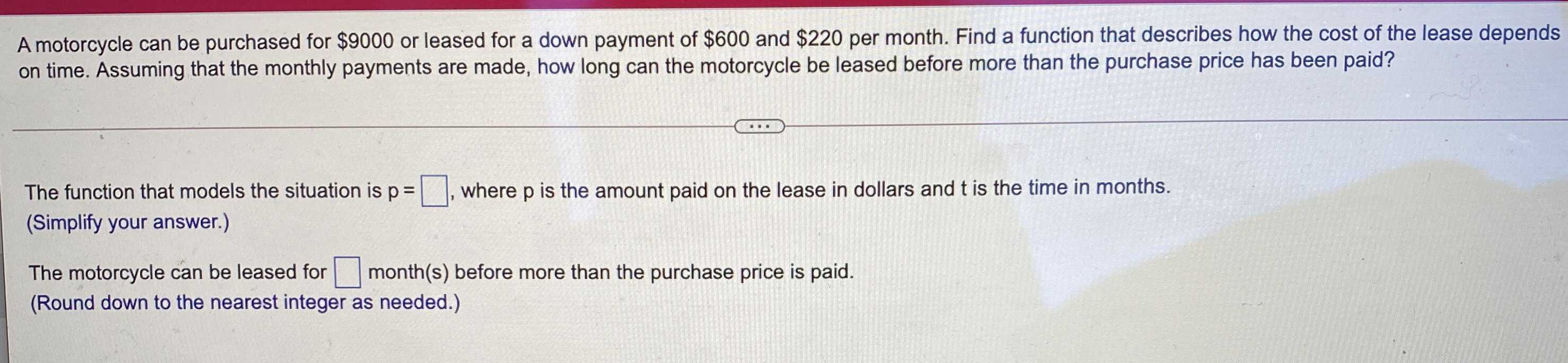### ¿Todavía tienes preguntas de matemáticas?

Pregunte a nuestros tutores expertos
Algebra
PreguntaA motorcycle can be purchased for $$\ 9000$$or leased for a down payment of $$\ 600$$and $$\ 220$$per month. Find a function that describes how the cost of the lease depends on time. Assuming that the monthly payments are made, how long can the motorcycle be leased before more than the purchase price has been paid?

The function that models the situation is $$p = \square$$, where $$p$$is the amount paid on the lease in dollars and $$t$$is the time in months. (Simplify your answer.)

The motorcycle can be leased for $$\square$$month(s) before more than the purchase price is paid.

(Round down to the nearest integer as needed.)